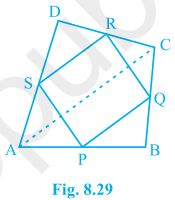# ABCD is a quadrilateral in which P, Q, R and S are mid-points of the sides AB, BC, CD and DA (see Fig) . AC is a diagonal. Show that: (i) SR || AC and SR = 1/2 AC (ii) PQ = SR (iii) PQRS is a parallelogram.Given

P, Q, R and S are the mid points of quadrilateral ABCD

Solution

Theorem :The line segment joining the mid-points of two sides of a triangle is parallel to the third side.

(i)  SR || AC and SR = 1/2 AC

Considering ∆ACD

we observe that S and R are the mid points of side AD and DC respectively. [Given]

Hence,SR || AC and SR = ½ AC (As per the above theorem)…………………….(1)

(ii) PQ = SR

Considering ∆ACB

We observe that P and Q are the mid points of side AB and BC respectively. [Given]

Hence, PQ || AC and PQ = ½ AC (As per above theorem)…………………………(2)

From (1) and (2) we can say,

PQ = SR

(iii) PQRS is a parallelogram

rom (i) and (ii) we can say that

PQ || AC and SR || AC

so, PQ || SR and PQ = SR

If each pair of opposite sides of a quadrilateral is equal, then it is a parallelogram.

Hence, PQRS is a parallelogram.(54)(11)